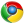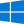# 正态分布

$$f(x) = \frac{1}{\sqrt{2\pi}\sigma} e^{-\frac{(x-\mu)^2}{2\sigma^2}}$$

• $P(-1.96 \leq Z \leq 1.96)$ = $P(-1.96 \leq \frac{X-\mu}{\sigma} \leq 1.96)$ = 0.95
• $P(-2 \leq Z \leq 2)$ = $P(-2 \leq \frac{X-\mu}{\sigma} \leq 2)$ = 0.9545
• $P(-3 \leq Z \leq 3)$ = $P(-3 \leq \frac{X-\mu}{\sigma} \leq 3)$ = 0.9973

$$Z = \frac{X - \mu}{\sigma}$$

1.MapMaths说道：Google ChromeWindows 10
MathJax又炸了（）
1.C1en说道：Google ChromeWindows 10
难用死了(((过几天有时间自己写一个库
2.MapMaths说道：Google ChromeWindows 10
好神奇，头像是看的邮箱的吗？
1.C1en说道：Google ChromeWindows 10
Gravatar啊(